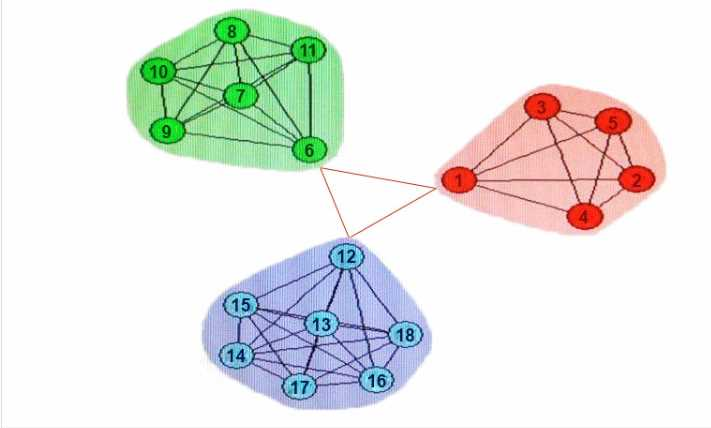# Dell-EMC e20-065 practice test

## Data Scientist Advanced Analytics Specialist Exam

##### Question 1

What is the maximum degree of a node in an undirected graph with 50 nodes'?

• A. 49
• B. 50
• C. 1250
• D. 2500

A

Discussions
0 / 600

##### Question 2

What is NOT a category of a NoSQL data store?

• A. Columnar
• B. Document
• C. Key/Value
• D. Flat File

D

Discussions
0 / 600

##### Question 3

A hotel chain runs a simul-ation on room pricing. They want to estimate revenue, per hotel, within
+/- \$10 with 95% confidence (Za/2=1.96). The estimated revenue standard deviation is \$5000 based
on previous booking data.
What is the optimal number of simulation trials to run?

• A. A 32-bit operating system was used
• B. The same number of trials was used
• C. A linear congruential generator (LCG) was used (or pseudo-random number generation
• D. Different seeds tor the random number generator were used.

C

Discussions
0 / 600

##### Question 4

A simul-ation to compare two different sales models yields different results for the same set of input
variables in different runs.
What is the likely cause?

• A. bit operating system was used
• B. The same number of trials was used.
• C. A linear congruenlial generator (LCG) was used for pseudo-random number generation.
• D. Different seeds forthe random number generator were used

C

Discussions
0 / 600

##### Question 5

A data engineer is asked to process several large datasets using MapReduce. Upon initial inspection
the engineer realizes that there are complex interdependencies between the datasets.
Why is this a problem?

• A. MapReduce works best on unstructured data
• B. There is no problem; MapReduce accommodates all the data
• C. MapReduce can only parse one file at a time.
• D. MapReduce is not ideal when the processing of one dataset depends on another.

D

Discussions
0 / 600

##### Question 6

What is a key beneficial characteristic of the Random Forest algorithm?

• A. Provides and explanatory model
• B. Distinguishes categorical from continuous variables
• C. Support for unstructured data
• D. Resiliency to complex, non-linear variable interactions

D

Discussions
0 / 600

##### Question 7

If two of the communities are re-designated to be one community, how does that change the
network characteristics?
Refer to the exhibit.• A. Neighborhood overlap would increase
• B. Network diameter would decrease
• C. Modularity would increase
• D. Modularity would decrease

B

Discussions
0 / 600

##### Question 8

What is a characteristic of stemming?

• A. Reduces words of variant forms to their base forms based on a set of heuristics
• B. Can be performed by calling the stemming!) function on a lemma in NLTK
• C. Can be performed by calling the stemming() function on a synset in NLTK
• D. Reduces words of variant forms to their base forms based on a dictionary

A

Discussions
0 / 600

##### Question 9

What does YARN provide over and above MapReduce?

• A. Separate cluster and resource management
• B. Parallelized processing
• C. Serialized processing

A

Discussions
0 / 600

##### Question 10

Which Hadoop Files System shell command copies data from a local file system into HDFS?

• A. rm
• B. cp
• C. put
• D. get